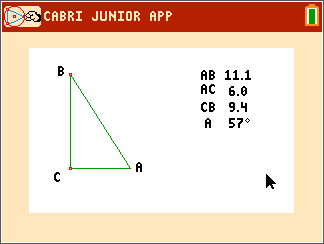### Mathematics lessons for IB® Diploma Programme

Applications and Interpretation | Geometry and Trigonometry

# Trig Ratios

### Objectives• Students will understand that trigonometric functions of an angle do not depend on the size of the triangle within which the angle is contained, but rather on the ratios of the sides of the triangle.
• Students will understand the meaning of reference angles and use reference angles to determine the trigonometric functions of a given angle.
• Students will create an algorithm to determine the trigonometric functions of an angle based on its reference angle.

### Vocabulary

• logarithmic expressions
• equivalent expressions
• exponential properties